# ML Aggarwal Class 6 Solutions for ICSE Maths Chapter 7 Decimals Check Your Progress

## ML Aggarwal Class 6 Solutions for ICSE Maths Chapter 7 Decimals Check Your Progress

Question 1.
Convert the following decimal numbers into fractions (in lowest terms).
(i) 6.015
(ii) 0.876
(iii) 23.375
Solution: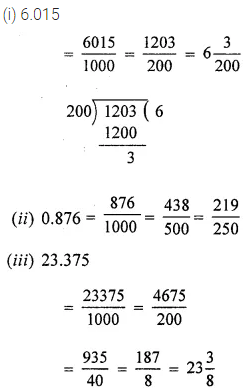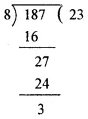Question 2.
Write the following fractions as decimals numbers:Solution:Question 3.
Arrange the following decimal numbers in ascending order:
(i) 123.8, 74.205, 74.209, 7.4209
(ii) 85.01, 85.1, 85.001, 85.103
Solution: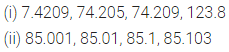Question 4.
Arrange the following decimal numbers in desending order:
(i) 6.45, 4.65, 6.405, 64.5, 6.54
(ii) 73.5, 35.7, 7.35, 7.53, 7.035
Solution: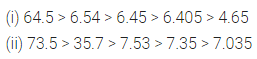Question 5.
If the schoolbags of Garima and Nakul weigh 5.2 kg and 4.832 kg respectively, find
(i) the total weight.
(ii) the difference in weight of the bags.
Solution: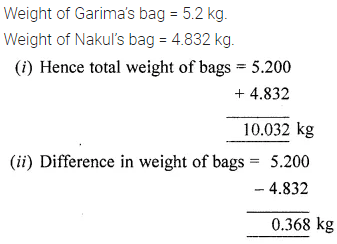Question 6.
Evaluate the following:
(i) 31.42 – 17.853 -6.43
(ii) 13.01 – 5.428 – 3.703 + 2.99.
Solution:Question 7.
By how much does the sum of 15.453 and 31.647 exceed the sum of 18.47 and 19.506?
Solution: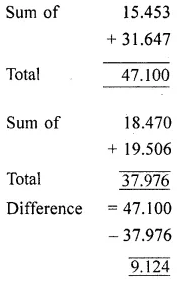Question 8.
Namita travels 20 km 50 m every day. Out of this she travels 10 km 200 m by bus and the rest by auto. How much distance does she travel by auto?
Solution: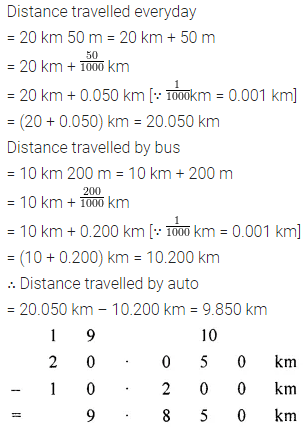Question 9.
Ravi purchased 5 kg 400 g rice, 2 kg 20 g sugar and 10 kg 850 g flour (aata). Find the total weight of his purchases.
Solution:Question 10.
1 kg of pure milk contains 0.263 kg of fat. How much fat is there 15.5 kg of milk?
Solution: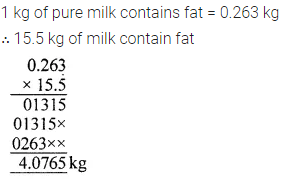Question 11.
The product of two numbers is 15.275. If one number is 4.7, find the other.
Solution: